# Stiffness Method for Beams with examples

Before going into the detailed Stiffness Method for Beams, let’s discuss some basic concepts and the general matrix methods of analysis for indeterminate structures.

## Matrix Methods of Analysis

Matrix analysis of a structure is a branch of structural analysis in which matrix algebra is used as a tool for structural analysis.
The aim of the structure analysis is to predict the behavior of a structure due to external loads, temperature changes, settlement of supports, vibrations, etc.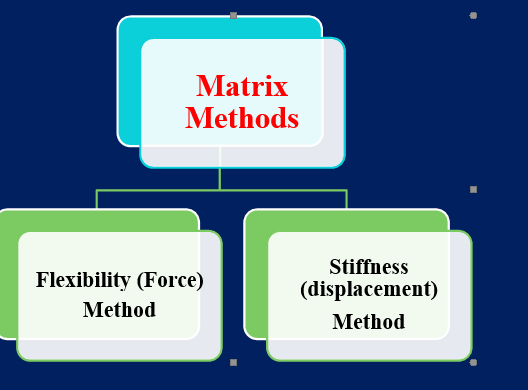## Flexibility Method:

With this method, redundant restrictions are removed, and corresponding redundant actions (forces or moments) are placed. A compatibility equation for the deformation is written from these redundant and corresponding displacements.

The redundancies are determined from these simultaneous equations. Static equations are then used to calculate the dimensioned internal actions. In this method, actions are treated as fundamental unknowns.

## Stiffness or Displacement Method

The displacement method of analysis writes the equilibrium equations in the form of the unknown joint displacements in terms of loads using load-displacement relationships.
The unknown displacements of the joints (the degrees of freedom of the structure) are calculated by solving simultaneous equilibrium equations.
The displacement analysis method is preferred over the computer implementation.

Stiffness:
The stiffness of a rod measures its resistance to deformation. The stiffness of an element is defined as the force that must be applied at a given point to produce a unit displacement when all other displacements are constrained to be zero.

If an elastically behaving rod is exposed to an alternating axial tensile load (W), as shown in Fig.5.1 and a graph is drawn of load (W) versus displacement () the result will be a straight line as shown in fig. 5.2, stiffness is the slope of this line.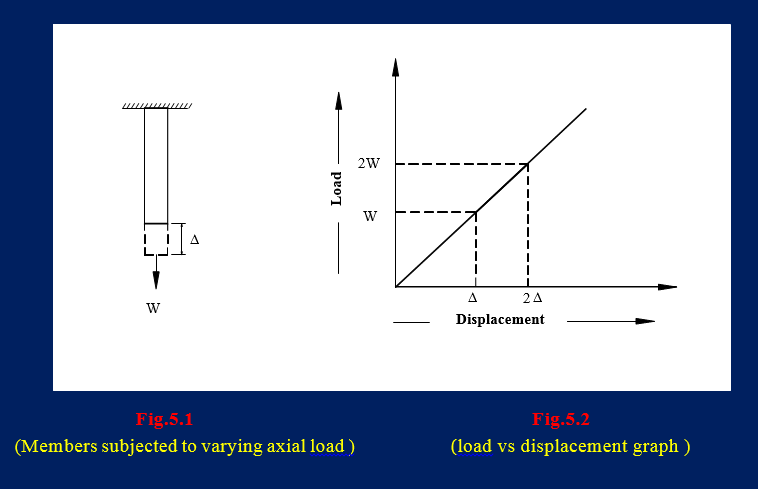Mathematically it can be expressed as: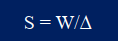In other words, the stiffness “S” is the force required at a given point to cause the unit to move at that point.

We can write the above equation in the following form: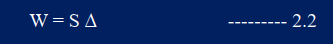Where,
W = force at a given point
S = stiffness (k)
Delta = unit shift of the respective point.

The above equation relates to force and displacement at a single point. This can be extended to develop a relationship between load and displacement for more than one point of a structure.

## Relationship between flexibility & stiffness:

Since the spring is linearly elastic i.e. actions and displacements are directly proportional i.e.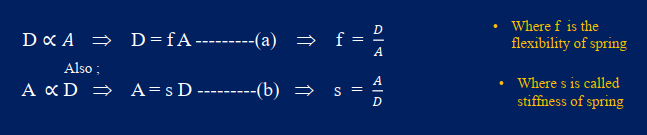## Kinematic indeterminacy:

The degree of kinematic indeterminacy of a structure is the number of unconstrained degrees of freedom (displacements).
A structure is said to be kinematically indeterminate if the displacement components of its joints cannot be determined completely by compatibility conditions. i.e. no. of unknown joint displacements beyond the compatibility conditions result in the degree of kinematic uncertainty.

Generally for a planer structure

𝐾. 𝐼 = 3𝑗 — 𝑟 + 𝑖
where

j = no. of joints
r = no. of reactions
i = no. of internal hinges.

Ignoring axial deformation ⇒ if we ignore axial deformation then the kinematic indeterminacy will decrease. (why we are ignoring axial deformations? The Kinematic indeterminacy of the structure after ignoring the axial deformation is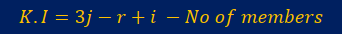## Kinematic indeterminacy for 2 dimension

The fixed column gives 3 reactions, one rotation, and 2 translations, therefore the degree of freedom for fixed columns is 0.
• Hinge gives 2 reactions both translations and no rotation reaction hence the degree of freedom at the hinge is 1, rotation.
• The Roller support gives 1 reaction in translation direction thus, the degree of freedom at roller is 2, that is one rotation and one translation.
• For a 2 dimension rigid joint or free end the number of degrees of freedom at a joint is 3 (one rotation and 2 translations).
• For the discontinuity-like internal hinge, the degree of freedom is 4, two rotational and 2 translational.

## Kinematic indeterminacy for 3 dimension

Fixed support gives 6 reactions, 3 rotations, and 3 translations, therefore the degree of freedom with fixed support is 0,
• Hinge gives 3 reactions all in translations and no rotation reaction hence the degree of freedom at the hinge is 3, rotation in all directions.
• The roller gives 1 reaction when translating in one direction, therefore the degree of freedom on the roller is 5, 3 revolutions, and 2 translations.

• For a 3 dimension rigid joint or free end the number of degrees of freedom at a joint is 6 (3 rotations and 3 translations).
• For the internal hinge, the degree of freedom is 9, 6 rotations, and 3 translations.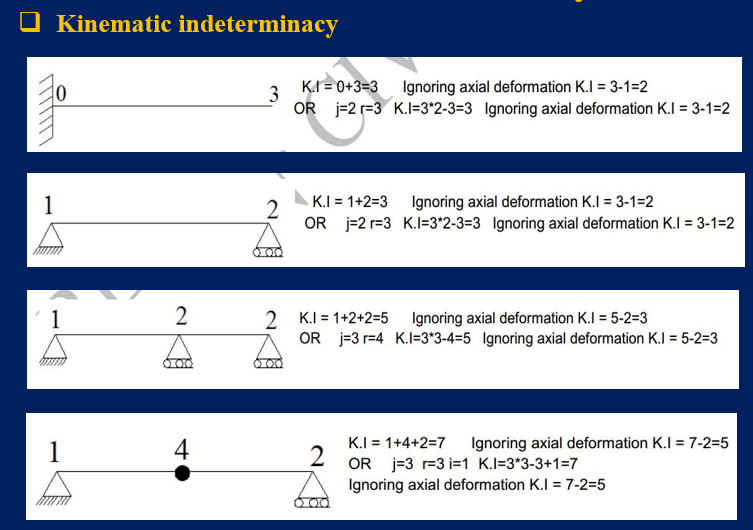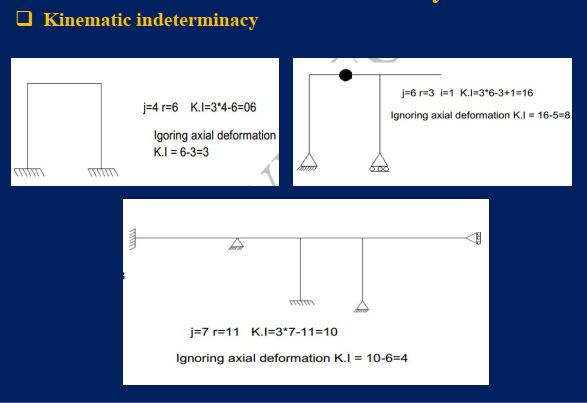## The general procedure of Stiffness Method for Beams

In this method, the degree of K.I is determined and the displacement components are identified.

     A coordinate is assigned to each independent displacement component. Thus D1, D2, D3……….Dn is the redundant displacement at 1,2,3……….n locations.

     If all the redundant displacements restrained the resultant structure known as Restrained structure or Basic kinematically determinate structure.

It first requires the fulfillment of equilibrium equations for the structure. That’s why the unknown displacements are compactly written in the form of the loads via the load-displacement relationships and these equations are then solved for the unknown displacements.

After the determination of the displacement, the unknown loads are determined from the compatibility equations by using the well-known load-displacement relationships. Thus, For n independent displacements.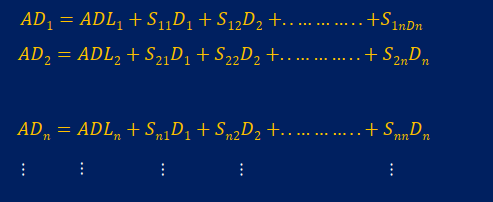Writing the equations on the previous slide in matrix form is known as the matrix formulation of the stiffness method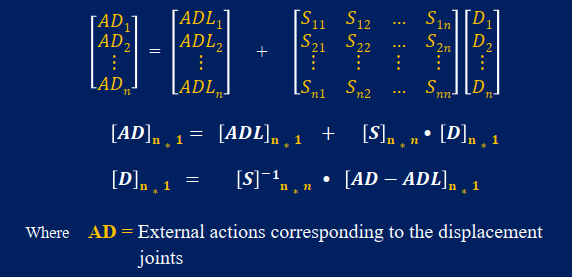ADL = Actions corresponding to the displacement joints in the restrained structure due to applied loads.( Fixed end actions) D = The unknown joint displacements S = Stiffness coefficient i.e displacement per unit force/action Step-wise Solution Procedure using Stiffness method:

• Step # 01: Make the structure kinematically determinate, by restraining the joints i.e. select the redundant joint displacement.

• Step # 02: Now apply the actual loading as given in the problem on the BKDS (Basic kinematically determinate structure) and find the resulting actions at the locations of redundant joints ( calculate the fixed end actions) this will generate an ADL matrix.

• Step # 03: Apply the redundant joint displacement on the BKDS (To standardize the procedure, only a unit displacement is applied in the +ve direction) this will generate a stiffness coefficient matrix.

• Step # 04: Now apply and write the equilibrium equations and conditions at the location of the redundant joint displacement to solve for unknown joint displacement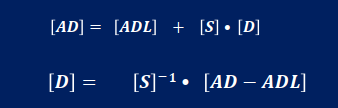• Step # 05: Finally Compute the member end actions and draw the shear and bending force diagram.

## Example: 01

Let’s illustrate the above-mentioned procedure with the help of a general example.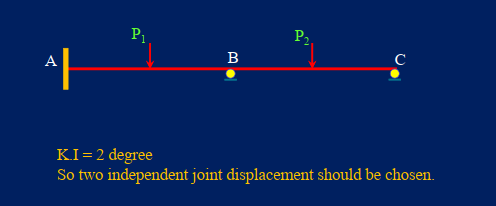• Step # 01: In the first step Select redundant Joint displacements and assign coordinates at those locations. Also, compute AD values.

• Step # 02: Then the restrained structure is acted upon by the actual applied loads. Calculate the values of the actions in the restrained structure that correspond to the redundant places. This will generate the structure ADL matrix. ( Fixed end actions)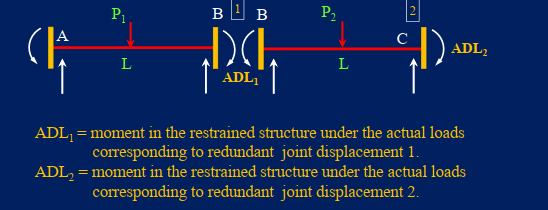Step # 03: Apply a unit value of D on the BKDS & calculate the resulting stiffness coefficient values in the BKDS that correspond to the redundant joint displacement points.
• First, a unit rotation is applied at position 1 and prevented at position 2 as shown. Now Calculate the values of S11 and S21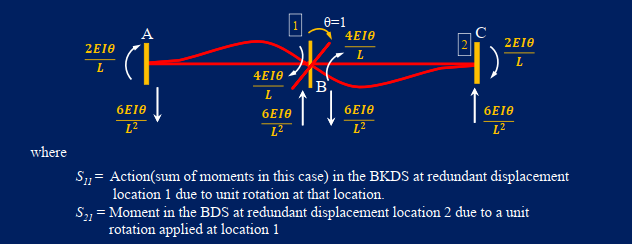Apply a unit rotation at the redundant displacement location * 2 and prevent it at 1 as shown. Finally, Calculate the values of S12 & S22.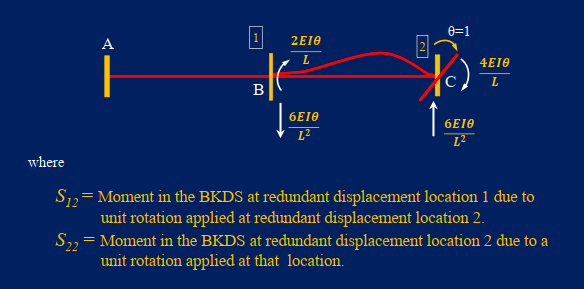Step # 04: Applying an equilibrium condition at the location of the redundant joint displacement to write equilibrium equations and solve for unknown joint displacement.Step # 05: Calculate the element end actions, same procedure as described in the flexibility method.
Member endings: Member endings are the moments and shear at each point that they are changed. We can determine the member end effects with the stiffness method if the redundant joint displacements are known.
• Let’s illustrate the process of finding member actions by the Rigidity method using the above example as shown in Fig. 1.Step # 02: Determine the member end effects (shear & moments) in the restrained structure under the actually applied loads (AMLn) at the specified locations.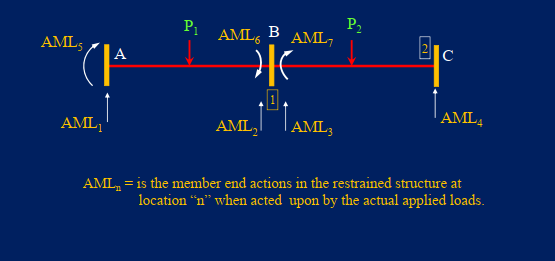Step # 03: Determine the member end effects (shear & moments) in the restrained structure if a unit acts during displacement 1. at redundant displacement point 1 and then at 2 (AMDn1 & AMDn2).
When a unit action is applied at redundant site 1 as shown.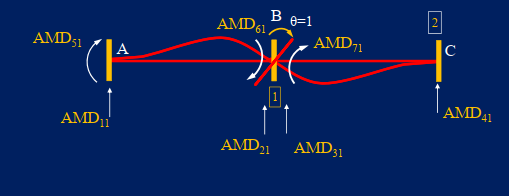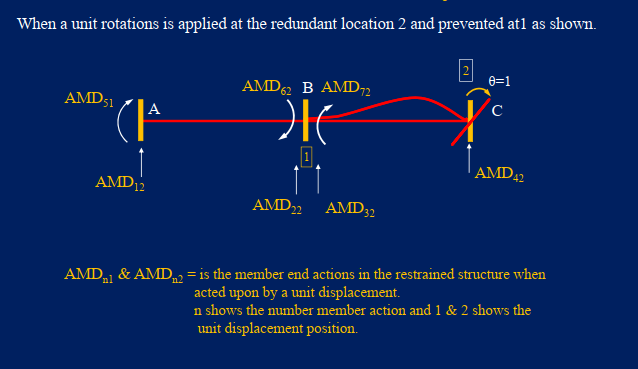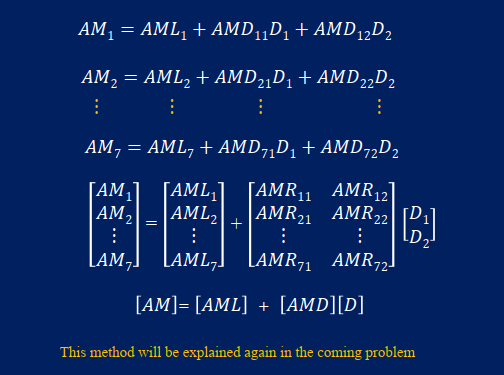Some frequently asked questions about the stiffness method are discussed below:

### Stiffness method is used to calculate

The stiffness method is used to calculate the beam’s deflection. This method is also known as the energy method. To calculate the deflection, we use the elastic and potential energies of the system. Using the virtual work principle, we can write the deflection equation.

Using this method, we can calculate the deflection at any point on the beam.

### The stiffness of a structure is defined as

The ability of a structural element to resist deflection or deformation when subjected to an applied force is referred to as a building’s stiffness. In general, this refers to how well the element resists bending or displacement under the influence of a supplied force.

## Conclusion: Stiffness Method

The stiffness method is a very effective matrix method used for the analysis of indeterminate structures. The overall procedure is to solve the structure using matrix algebra, which is then very easy to solve through computer programs. Excel sheets prepared for the stiffness method for beams can really help in this regard.